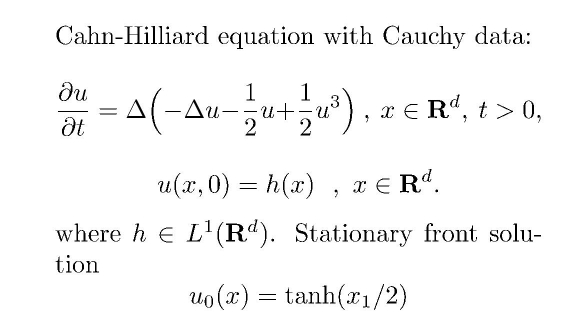Main page Department of Mathematics and Statistics of University of HelsinkiFormula 1. Cahn-Hilliard-equation.Formula 2. The parabolic equation for the perturbation.The Cahn-Hilliard equation (Formula 1) can be used to model phase separation in mixtures of two substances A and B: the function u has values in the interval [-1,1] with the endpoints describing the state of pure phase of substance A, respectively, B. For a random initial distribution h, numerical simulations show that phase segregation evolves: domains of phase A and B start to form. However, a rigorous mathematical analysis of this phenomenon seems very difficult. SOME RECENT ARTICLES T.Korvola, A.Kupiainen, J.Taskinen: Anomalous scaling for 3d Cahn-Hilliard fronts Comm. Pure Appl. Math. LVIII, (2005), 1077-1115. This work deals with the simple situation that the initial data is a perturbation of the stationary kink solution tanh (x/2) for the unbounded Euclidean space of at least dimension 3. We prove the stability: the function u tends, as expected, to the stationary solution, if the initial perturbation is small enough. The method is based on a detailed spectral analysis of the linear elliptic fourth order operator L, see Formula 2 (using the Melnikov, or Pego-Weinstein, method), and a fixed point argument in some properly chosen Banach spaces with weighted sup-norms. J.Bricmont, A.Kupiainen, J.Taskinen: Stability of Cahn-Hilliard fronts Comm.Pure.Appl.Math. LII (1999), 839-871. This earlier work proves the stability of the stationary solution in the case x ∈ R. The approach is based on the renormalization group method. Back to main page .